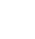# Add every other cell on a row

Occasionally when you have a large number of cells in a row that you want to sum, ideally it would just be in a range. ie A1:A7500 for instance. This would sum up everything from Cell A1 to Cell A7500.

Now what if the data contained daily takings where 1 column was Gross and the next was Net then the next one was Gross and then Net and so on ? A formula to add every other one might look like this =SUM(A1+A3+A5+…..A351+A353+….A7500). This would take hours!

A formula that we use at Datanology solves this quite easily:

``` =SUMPRODUCT(--(MOD(COLUMN(E1:N1)-COLUMN(D1)+1,2)=0),E1:N1) ```

This will sum every other column starting at E1 right up to N1. The middle part that refers to D1 tells it where to start, the =0 part tells it to do even columns ie 2nd,4th,6th,8th, etc.